## NCERT Solutions for Class 10 Maths Chapter 5 Arithmetic Progressions Ex 5.3

NCERT Solutions for Class 10 Maths Chapter 5 Arithmetic Progressions Ex 5.3 are part of NCERT Solutions for Class 10 Maths. Here we have given NCERT Solutions for Class 10 Maths Chapter 5 Arithmetic Progressions Ex 5.3. https://mcqquestions.guru/ncert-solutions-for-class-10-maths-chapter-5-ex-5-3/

 Board CBSE Textbook NCERT Class Class 10 Subject Maths Chapter Chapter 5 Chapter Name Arithmetic Progressions Exercise Ex 5.3 Number of Questions Solved 20 Category NCERT Solutions

## NCERT Solutions for Class 10 Maths Chapter 5 Arithmetic Progressions Ex 5.3

Ex 5.3 Class 10 Maths Question 1.
Find the sum of the following APs:
(i) 2, 7, 12,…… to 10 terms.
(ii) -37, -33, -29, …… to 12 terms.
(iii) 0.6, 1.7, 2.8, ……, to 100 terms.
(iv) $$\frac { 1 }{ 15 }$$, $$\frac { 1 }{ 12 }$$, $$\frac { 1 }{ 10 }$$, …….., to 11 terms.
Solution: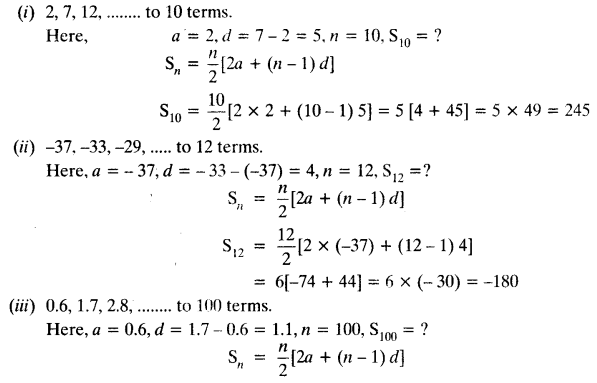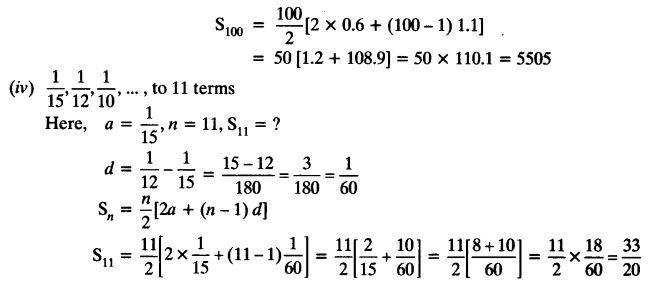Exercise 5.3 Class 10 Maths Question 2.
Find the sums given below:
(i) 7 + 10$$\frac { 1 }{ 2 }$$ + 14 + … + 84
(ii) 34 + 32 + 30 + … + 10
(iii) -5 + (-8) + (-11) + ….. + (-230)
Solution: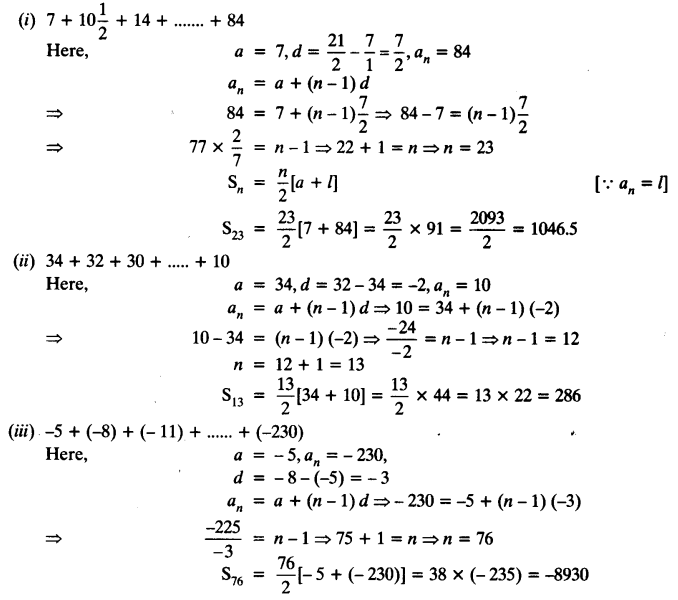5.3 Class 10 Maths Question 3.
In an AP:
(i) given a = 5, d = 3, an = 50, find n and Sn.
(ii) given a = 7, a13 = 35, find d and S13.
(iii) given a12 = 37, d = 3, find a and S12.
(iv) given a3 = -15, S10 = 125, find d and a10.
(v) given d = 5, S9 = 75, find a and a9.
(vi) given a = 2, d = 8, Sn = 90, find n and an.
(vii) given a = 8, an = 62, Sn = 210, find n and d.
(viii) given an = 4, d = 2, Sn = -14, find n and a.
(ix) given a = 3, n = 8, S = 192, find d.
(x) given l = 28, S = 144, and there are total 9 terms. Find a.
Solution: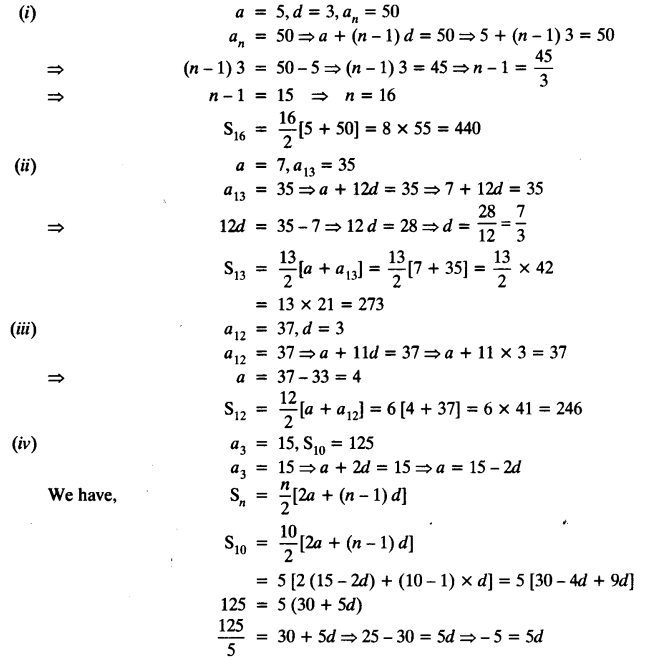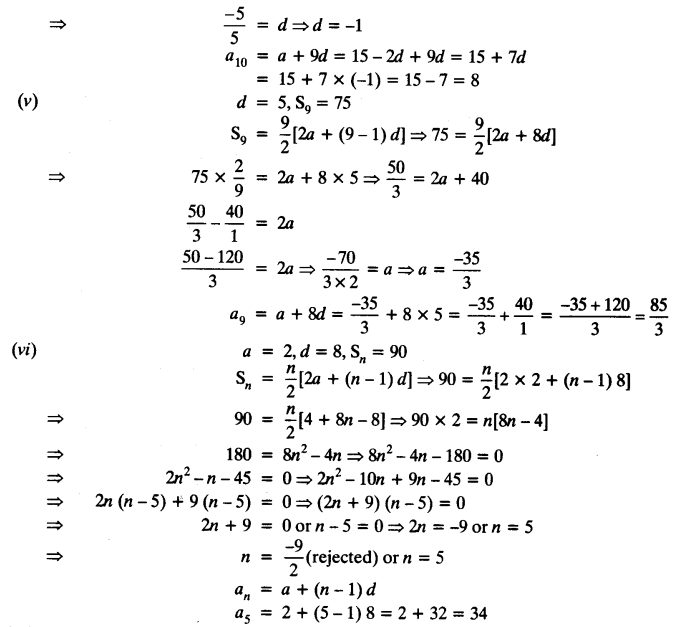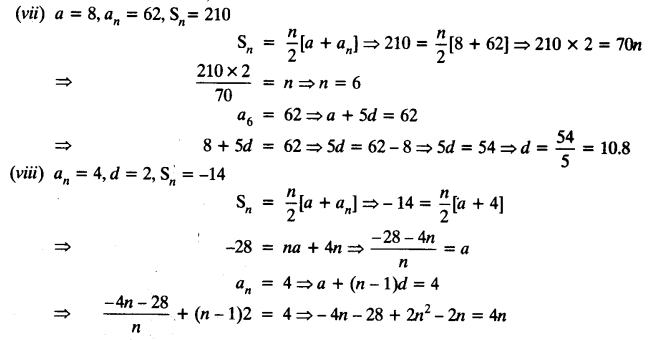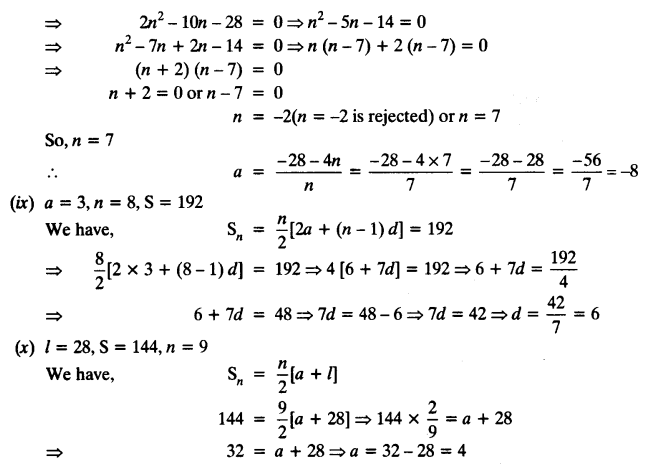Ex5.3 Class 10 Maths Question 4.
How many terms of AP: 9, 17, 25, … must be taken to give a sum of 636?
Solution: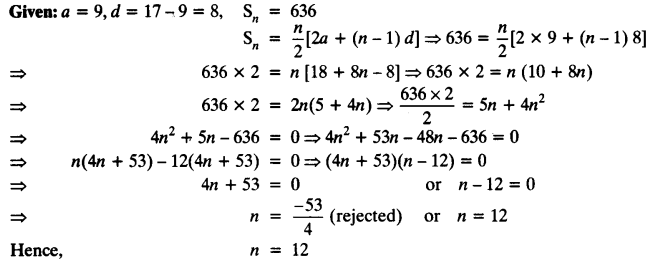Class 10 Ex 5.3 Maths Question 5.
The first term of an AP is 5, the last term is 45 and the sum is 400. Find the number of terms and the common difference.
Solution: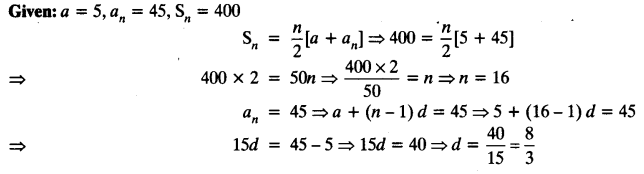Class 10 Maths Chapter 5 Exercise 5.3 Question 6.
The first and the last terms of an AP are 17 and 350 respectively. If the common difference is 9, how many terms are there and what is their sum?
Solution: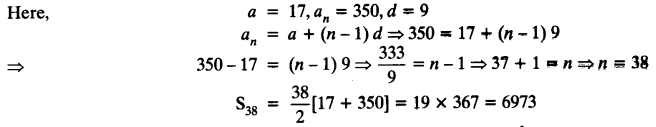Ex 5.3 Maths Class 10 Question 7.
Find the sum of first 22 terms of an AP in which d = 7 and 22nd term is 149.
Solution: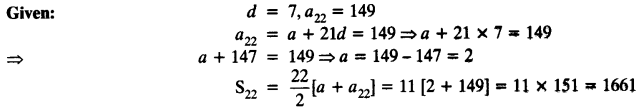5.3 Maths Class 10 Question 8.
Find the sum of first 51 terms of an AP whose second and third terms are 14 and 18 respectively.
Solution: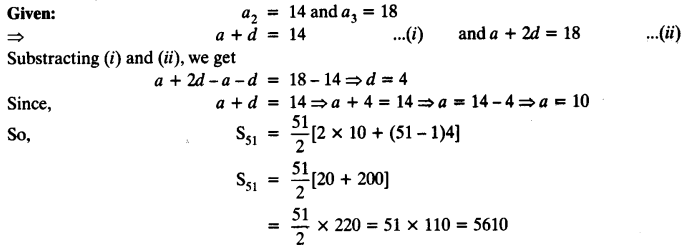Exercise 5.3 Maths Class 10 Question 9.
If the sum of first 7 terms of an AP is 49 and that of 17 terms is 289, find the sum of first n terms.
Solution: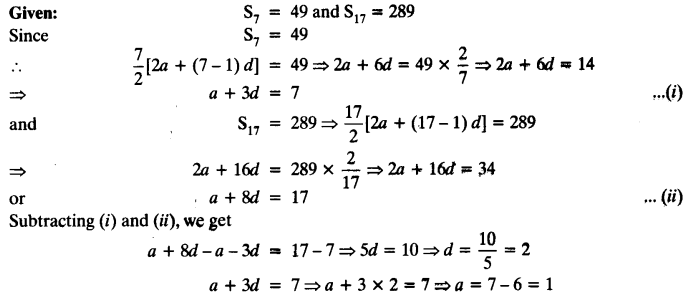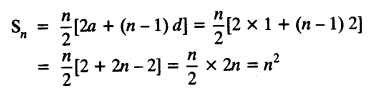Class 10 Maths Ex 5.3 Question 10.
Show that a1, a2, ……. an,…… form an AP where an is defined as below:
(i) an = 3 + 4n
(ii) an = 9 – 5n
Also find the sum of the first 15 terms in each case.
Solution: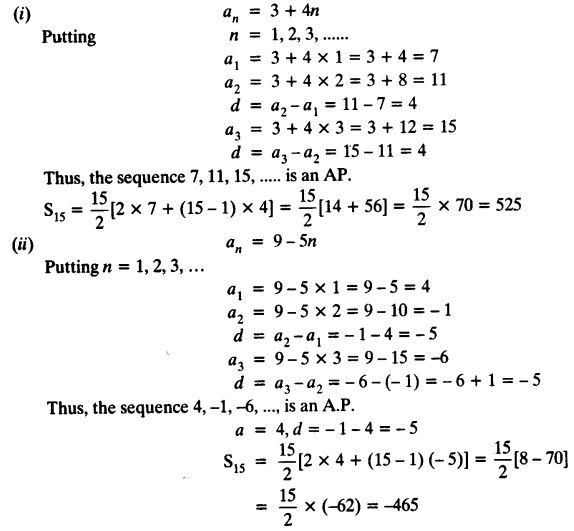Ncert Solutions For Class 10 Maths Chapter 5 Exercise 5.3 Question 11.
If the sum of the first n terms of an AP is 4n – n2, what is the first term (that is S1)? What is the sum of first two terms? What is the second term? Similarly, find the 3rd, the 10th and the nth terms.
Solution: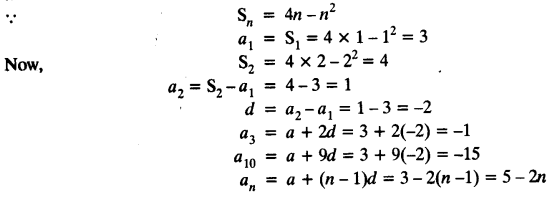Class 10th Exercise 5.3 Question 12.
Find the sum of the first 40 positive integers divisible by 6.
Solution: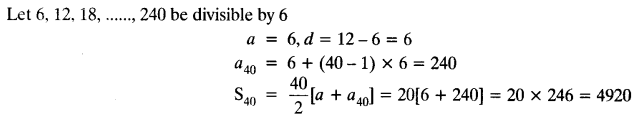Exercise 5.3 Class 10 Solutions Question 13.
Find the sum of the first 15 multiples of 8.
Solution: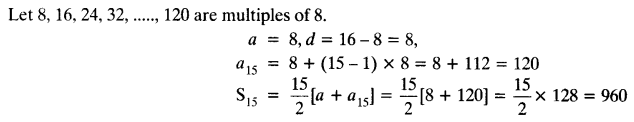Exercise 5.3 Class 10th Question 14.
Find the sum of the odd numbers between 0 and 50.
Solution:
Let odd numbers between 0 and 50 be 1, 3, 5, 7,…….., 49.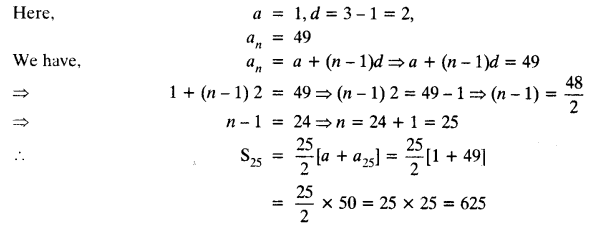Class 10 Maths Chapter 5 Exercise 5.3 Solution Question 15.
A contract on construction job specifies a penalty for delay of completion beyond a certain date as follows:
₹ 200 for the first day, ₹ 250 for the second day, ₹ 300 for the third day, etc. the penalty for each succeeding day being ₹ 50 more than for the preceding day. How much money the contractor has to pay as penalty, if he has delayed the work by 30 days?
Solution: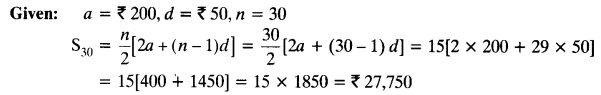Class 10 Maths Ch 5 Ex 5.3 Chapter 5 Question 16.
A sum of ₹ 700 is to be used to give seven cash prizes to students of a school for their overall academic performance. If each prize is ₹ 20 less than its preceding prize, find the value of each of the prizes.
Solution:
Let 1st prize be of ₹ a
2nd prize be ₹ (a – 20) and
3rd prize be ₹ (a – 20 – 20) = ₹ (a – 40)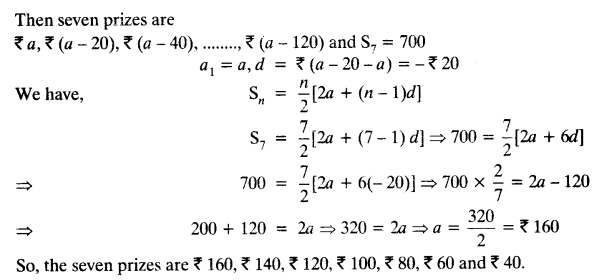Class 10 Chapter 5 Exercise 5.3 Question 17.
In a school, students thought of planting trees in and around the school to reduce air pollution. It was decided that the number of trees, that each section of each class will plant, will be the same as the class, in which they are studying, eg. a section of Class I will plant 1 tree, a section of Class II will plant 2 trees and so on till Class XII. There are three sections of each class. How many trees will be planted by the students?
Solution:
Let the trees be planted 1, 2, 3, 4, 5 , …… 12
Here, a = 1, d = 1, n = 12
Total number of trees planted by each section
S12 = $$\frac { 12 }{ 2 }$$ [2a + (n – 1) d] = 6 [2 x 1 + (12 – 1) x 1]
= 6 [2 + 11] = 6 x 13 = 78
Total number of trees planted by 3 sections = 78 x 3 = 234

Class 10 Maths Chapter 5 Exercise 5.3 Solution Question 18.
A spiral is made up of successive semicircles, with centres alternately at A and B, starting with centre at A, of radii 0.5 cm, 1.0 cm, 1.5 cm, 2.0 cm,… as shown in figure. What is the total length of such a spiral made up of thirteen consecutive semicircles?
(Take π = $$\frac { 22 }{ 7 }$$)
[Hint: Length of successive semicircles is l1, l2, l3, l4, … with centres at A, B, respectively.]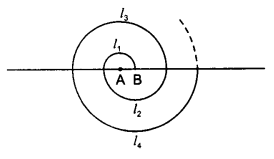Solution: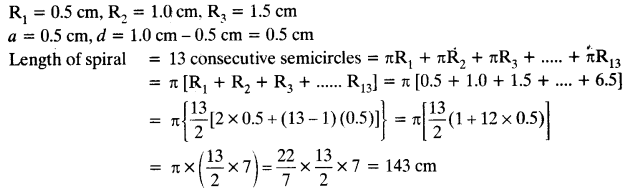5.3 Exercise Class 10 Question 19.
200 logs are stacked in the following manner 20 logs in the bottom row, 19 in the next row, 18 in the row next to it and so on (see Figure). In how many rows are the 200 logs placed and how many logs are in the top row?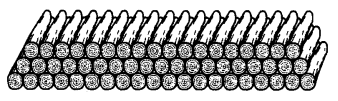Solution: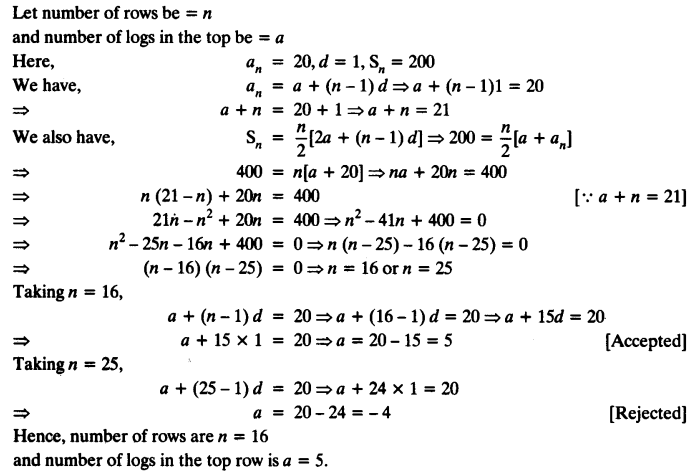Class 10th Exercise 5.3 Question 20.
In a potato race, a bucket is placed at the starting point, which is 5 m from the first potato, and the other potatoes are placed 3 m apart in a straight line. There are ten potatoes in the line (see Fig.) A competitor starts from the bucket, picks up the nearest potato, runs back with it, drops it in the bucket, runs back to pick up the next potato, runs to the bucket to drop it in, and she continues in the same way until all the potatoes are in the bucket. What is the total distance the competitor has to run?
[Hint: To pick up the first potato and the second potato, the total distance (in metres) run by a competitor is 2 x 5 + 2 x (5 + 3)]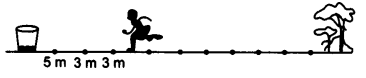Solution:
Distance between the first potato and the bucket = 5 m
Distance between next 2 potatoes = 3 m each
So, series is 5 m, 8 m, 11 m,
Here, a = 5 m, d = (8 – 5) m = 3 m
Total distance travelled for 10 potatoes = 2 [5 + 8 + 11 + …….. + 10 terms]
= 2[$$\frac { 10 }{ 2 }$${2 x 5 + (10 – 1) 3}]
= 2[5{10 + 27}] = 2(37 x 5) = 37 x 10 = 370 m.

We hope the NCERT Solutions for Class 10 Maths Chapter 5 Arithmetic Progressions Ex 5.3, help you. If you have any query regarding NCERT Solutions for Class 10 Maths Chapter 5 Arithmetic Progressions Ex 5.3, drop a comment below and we will get back to you at the earliest.

## NCERT Solutions for Class 10 Maths Chapter 4 Quadratic Equations Ex 4.4

NCERT Solutions for Class 10 Maths Chapter 4 Quadratic Equations Ex 4.4 are part of NCERT Solutions for Class 10 Maths. Here we have given NCERT Solutions for Class 10 Maths Chapter 4 Quadratic Equations Ex 4.4. https://mcqquestions.guru/ncert-solutions-for-class-10-maths-chapter-4-ex-4-4/

 Board CBSE Textbook NCERT Class Class 10 Subject Maths Chapter Chapter 4 Chapter Name Quadratic Equations Exercise Ex 4.4 Number of Questions Solved 5 Category NCERT Solutions

## NCERT Solutions for Class 10 Maths Chapter 4 Quadratic Equations Ex 4.4

Ex 4.4 Class 10 Maths Question 1.
Find the nature of the roots of the following quadratic equations. If the real roots exist, find them:
(i) 2x² -3x + 5 = 0
(ii) 3x2 – 4√3x + 4 = 0
(iii) 2x2-6x + 3 = 0
Solution:
(i) 2x2 – 3x + 5 = 0
This is of the form ax2 + bx + c = 0,
where a = 2, b = -3 and c = 5
Discriminant, D = b2-4ac
= (-3) 2-4 x 2 x 5 = 9 – 40 = -31
Since, D < 0
Hence, no real roots exist.

(ii) 3x2 – 4√3x + 4 = 0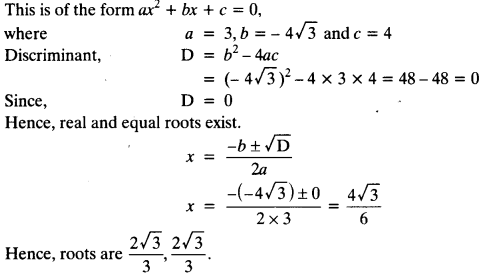(iii) 2x2-6x + 3 = 0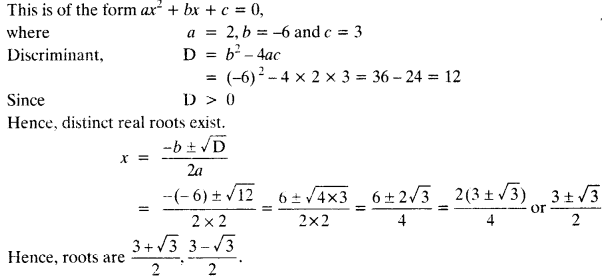4.4 Class 10 Maths Question 2.
Find the values of k for each of the following quadratic equations, so that they have two equal roots.
(1) 2x2 + kx + 3 = 0
(2) kx (x – 2) + 6 = 0
Solution: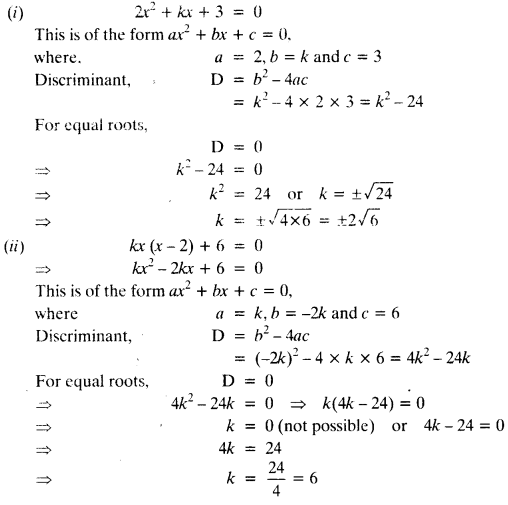Exercise 4.4 Class 10 Maths Question 3.
Is it possible to design a rectangular mango grove whose length is twice its breadth, and the area is 800 m2? If so, find its length and breadth.
Solution:
Let breadth of the rectangular mango grove be x m
Then, the length of rectangular mango grove be 2xm
According to question,
x x 2x = 800
⇒ 2x2 = 800
⇒ x2 = 400
⇒ x = ±20 [-20 is rejected]
Hence, breadth = 20 m and length = 2 x 20 = 40 m
So, it is possible to design a rectangular mango grove whose length is twice its breadth.

Ex4.4 Class 10 Maths Question 4.
Is the following situation possible? If so, determine their present ages.
The sum of the ages of two friends is 20 years. Four years ago, the product of their ages in years was 48.
Solution:
Let the present age of one friend be x years
Then, the present age of other friend be (20 -x) years
4 years ago, one friend’s age was (x – 4) years
4 years ago, other friend’s age was (20 -x – 4) = (16 -x) years
According to question,
(x-4) (16-x) = 48
⇒ 16x – x2 – 64 + 4x = 48
⇒ x2 – 20x + 112 = 0
This is of the form ax2 + bx + c = 0, where, a = 1, b = -20 and c = 112
Discriminant, D = b2 – 4ac
= (-20)2 – 4 x 1 x 112 = 400 – 448 = – 48 < 0
Since, no real roots exist.
So, the given situation is not possible.

Class 10 Maths Chapter 4 Exercise 4.4 Question 5.
Is it possible to design a rectangular park of perimeter 80 m and area 400 m2? If so, find its length and breadth.
Solution:
Let the length of rectangular park be x m and breadth be y m.
Given: area = 400 m2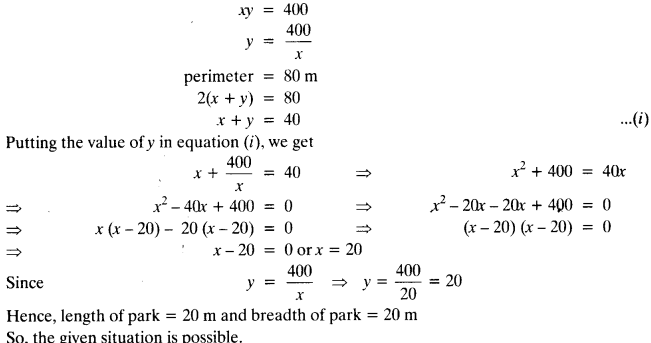We hope the NCERT Solutions for Class 10 Maths Chapter 4 Quadratic Equations Ex 4.4 help you. If you have any query regarding NCERT Solutions for Class 10 Maths Chapter 4 Quadratic Equations Ex 4.4, drop a comment below and we will get back to you at the earliest.

## NCERT Solutions for Class 10 Maths Chapter 3 Pair of Linear Equations in Two Variables Ex 3.7

NCERT Solutions for Class 10 Mathematics Chapter 3 Pair of Linear Equations in Two Variables Ex 3.7 are part of NCERT Solutions for Class 10 Maths. Here we have given NCERT Solutions for Class 10 Mathematics Chapter 3 Pair of Linear Equations in Two Variables Ex 3.7. https://mcqquestions.guru/ncert-solutions-for-class-10-maths-chapter-3-ex-3-7/

 Board CBSE Textbook NCERT Class Class 10 Subject Maths Chapter Chapter 3 Chapter Name Pair of Linear Equations in Two Variables Exercise Ex 3.7 Number of Questions Solved 8 Category NCERT Solutions

### NCERT Solutions for Class 10 Maths Chapter 3 Pair of Linear Equations in Two Variables Ex 3.7

Ex 3.7 Class 10 Maths Question 1.
The age of two friends Ani and Biju differ by 3 years. Ani’s father Dharam is twice as old as Ani and Biju is twice as old as his sister Cathy. The ages of Cathy and Dharam differ by 30 years. Find the ages of Ani and Biju.
Solution:
Let the ages of Ani and Biju be x years and y years respectively.
If Ani is older than Biju
x – y =3
If Biju is older than Ani
y – x = 3
-x + y =3   [Given]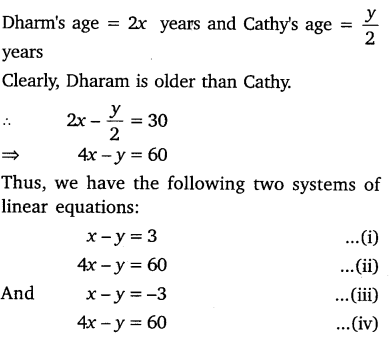Subtracting equation (i) from equation (ii), we get:
3x – 57
⇒ x = 19
Putting x = 19 in equation (i), we get
19-y = 3
⇒ y = 16
Again subtracting equation (iv) from equation (iii), we get
3x = 63
⇒  x =  21
Putting x = 21 in equation (iii) we get
21 -y=  -3
⇒  y  =   24
Hence, Ani’s age is   either 19 years or 21 years and Biju’s age is either 16 years or 24 years.

Exercise 3.7 Class 10 Maths Question 2.
One says, “Give me a hundred, friend! I shall then become twice as rich as you”. The other replies, “If you give me ten, I shall be six times as rich as you”. Tell me what is the amount of their (respective) capital?
Solution:
Let the two friends have ₹ x and ₹ y.
According to the first condition:
One friend has an amount = ₹(x + 100)
Other has an amount = ₹ (y – 100
∴  (x + 100) =2 (y – 100)
⇒  x + 100 = 2y – 200
⇒ x – 2y = -300       …(i)
According to the second condition:
One friend has an amount = ₹(x – 10)
Other friend has an amount =₹ (y + 10)
∴  6(x – 10) = y + 10
⇒ 6x – 60 = y + 10
⇒    6x-y = 70                                        …(ii)
Multiplying (ii) equation by 2 and subtracting the result from equation (i), we get:
x – 12x = – 300 – 140
⇒ -11x = -440
⇒  x = 40
Substituting x = 40 in equation (ii), we get
6 x 40 – y = 70
⇒ -y   = 70- 24
⇒  y   = 170
Thus, the two friends have ₹ 40 and ₹ 170.

3.7 Class 10 Maths Question 3.
A train covered a certain distance at a uniform speed. If the train would have been 10 km/h faster, it would have taken 2 hours less than the scheduled time. And, if the train were slower by 10 km/h, it would have taken 3 hours more than the scheduled time. Find the distance covered by the train.
Solution:
Let the original speed of the train be x km/h
and the time taken to complete the journey be y hours.            ‘
Then the distance covered = xy km

Case I: When speed = (x + 10) km/h and time taken = (y – 2) h
Distance = (x + 10) (y – 2) km
⇒   xy = (x + 10) (y – 2)
⇒ 10y – 2x = 20
⇒  5y – x = 10
⇒ -x + 5y = 10   …(i)

Case II: When speed = (x – 10) km/h and time taken = (y + 3) h
Distance = (x – 10) (y + 3) km
⇒  xy = (x – 10) (y + 3)
⇒ 3x- 10y = 30    …(ii)
Multiplying equation (i) by 3 and adding the result to equation (ii), we get
15y – 10y = 30 f 30
⇒ 5y = 60
⇒   y   = 12
Putting y = 12 in equation (ii), we get
3x- 10 x 12= 30
⇒  3x   = 150
⇒ x   = 50
∴  x = 50 and y =   12
Thus, original speed of train is 50 km/h and time taken by it is 12 h.
Distance covered by train = Speed x Time
=  50 x 12 = 600 km.

Ex 3.7 Maths Chapter 3 Question 4.
The students of a class are made to stand in rows. If 3 students are extra in a row, there would be 1 row less. If 3 students are less in a row, there would be 2 rows more. Find the number of students in the class.
Solution:
Let the number of rows be x and the number of students in each row be y.
Then the total number of students = xy
Case I: When there are 3 more students in each row
Then the number of students in a row = (y + 3)
and the number of rows = (x – 1)
Total number of students = (x – 1) (y + 3)
∴ (x – 1) (y + 3) = xy
⇒  3x  -y =3 …(i)
Case II: When 3 students are removed from each row
Then the number of students in each row = (y-3)
and the number of rows = (x + 2)
Total number of students = (x + 2) (y – 3)
∴  (x + 2) (y – 3) = xy
⇒ -3x + 2y = 6 …(ii)
Adding the equations (i) and (ii), we get
-y + 2y = 3 + 6
⇒ y = 9
Putting y = 9 in the equation (ii), we get
-3x +   18 = 6
⇒ x = 4
∴ x = 4 and y = 9
Hence, the total number of students in the class is 9 x 4 = 36.

Ex3.7 Class 10 Chapter 3 Maths Question 5.
In a ∆ABC, ∠C = 3 ∠B = 2(∠A + ∠B). Find the three angles.
Solution:
Let ∠A = x° and ∠B = y°.
Then ∠C = 3∠B = (3y)°.
Now ∠A + ∠B + ∠C = 180°
⇒ x + y + 3y = 180°
⇒ x + 4y = 180° …(i)
Also, ∠C = 2(∠A + ∠B)
⇒ 3y – 2(x + y)
⇒ 2x – y = 0° …(ii)
Multiplying (ii) by 4 and adding the result to equation (i), we get:
9x = 180°
⇒ x = 20°
Putting x = 20 in equation (i), we get:
20 + 4y = 180°
⇒ 4y = 160°
⇒  y =  $$\frac { 160 }{ 40 }$$  = 40°
∴ ∠A = 20°, ∠B = 40° and ∠C = 3 x 40° = 120°.

Class 10 Ex 3.7 Chapter 3 Maths Question 6.
Draw the graphs of the equations 5x – y = 5 and 3x – y = 3. Determine the coordinates of the vertices of the triangle formed by these lines and the y-axis.
Solution:
5x – y = 5    …(i)
3x-y = 3    …(ii)
For graphical representation:
From equation (i), we get: y = 5x – 5
When x = 0, then y -5
When x = 2, then y = 10 – 5 = 5
When x = 1, then y = 5 – 5 = 10
Thus, we have the following table of solutions: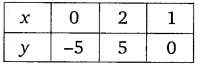From equation (ii), we get:
⇒ y = 3x – 3
When x = 0, then y = -3
When x = 2, then y = 6 – 3 = 3
When x = 1, then y = 3 – 3 = 0
Thus, we have the following table of solutions: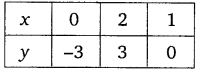Plotting the points of each table of solutions, we obtain the graphs of two lines intersecting each other at a point C(1, 0).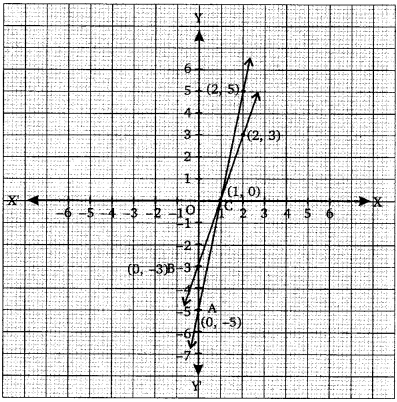The vertices of ΔABC formed by these lines and the y-axis are A(0, -5), B(0, -3) and C(1, 0).

Exercise 3.7 Class 10 Maths Chapter 3 Question 7.
Solve the following pairs of linear equations: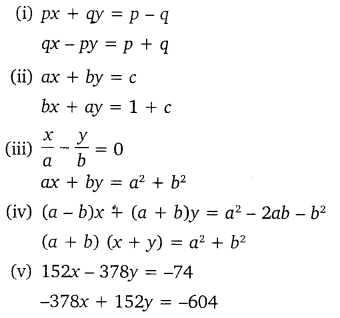Solution:
(i) The given equations are
px + qy = p – q  …(1)
qx – py = p + q …(2)
Multiplying equation (1) byp and equation (2) by q and then adding the results, we get:
x(p2 + q2) = p(p – q) + q(p + q)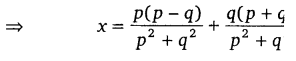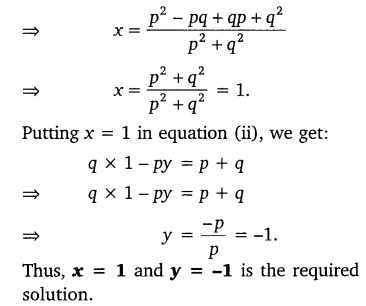(ii) The given equations are
ax + by = c  …(1)
bx – ay = 1 + c       …(2)
Multiplying equation (1) by b and equation (2) by a, we get:
abx + b2y = cb …(3)
abx + a2y = a(1+ c)  …(4)
Subtracting (3) from (4), we get: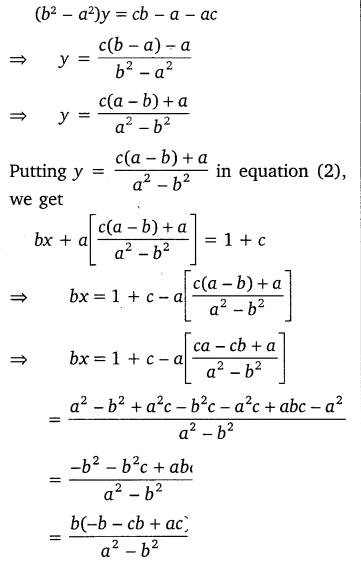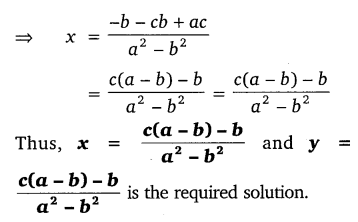(iii) The given equations may be written as: bx – ay = 0  …(1)
ax + by = a2 + b2   …(2)
Multiplying equation (1) by b and equation (2) by a, we get:
b2x + aby = 0 ….(3)
a2x + aby = a(a2 + b2) …..(4)
Adding equation (3) and equation (4), we get:
(a2 + b2)x = a (a2 + b2) a(a2 + b2)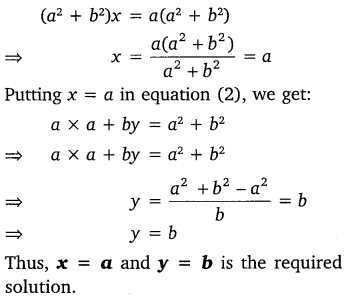(iv) The given equations may be written as:
(a – b)x + (a + b)y = a2 – 2ab – b2 …(1)
(a + b)x + (a + b)y = a2 + b2 …(2)
Subtracting equation (2) from equation (1), we get:
(a – b)x – (a + b)x
= (a2 – 2ab – b2) – (a2 + b2)
⇒ x(a – b- a-b) = a2 – 2ab – b2 – a2 – b2
⇒   -2bx = -2ab – 2b2
⇒ 2bx = 2b2 + 2ab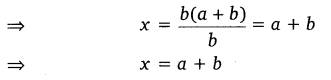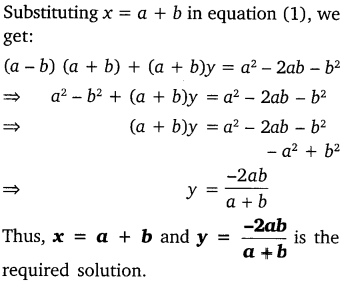(v) The given equations may be written as:
76x – 189y = -37 …(1)
-189x + 76y = -302   …(2)
Multiplying equation (1) by 76 and equation (2) by 189, we get:
5776x – 14364y = -2812  …(3)
-35721x + 14364y = -57078 …(4)
Adding equations (3) and (4), we get:
5776x – 35721x = -2812 – 57078
⇒ – 29945x = -59890
⇒  x = 2
Putting x = 2 in equation (1), we get:
76   x  2 – 189y   = -37
⇒ 152 – 189y   = -37
⇒ -189y  = -189
⇒  y = 1
Thus, x = 2 and y = 1 is the required solution.

Class 10 Maths Ex 3.7 Pair of Linear Equations in Two Variables Question 8.
ABCD is a cyclic quadrilateral (see figure). Find the angles of the cyclic quadrilateral.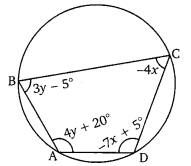Solution: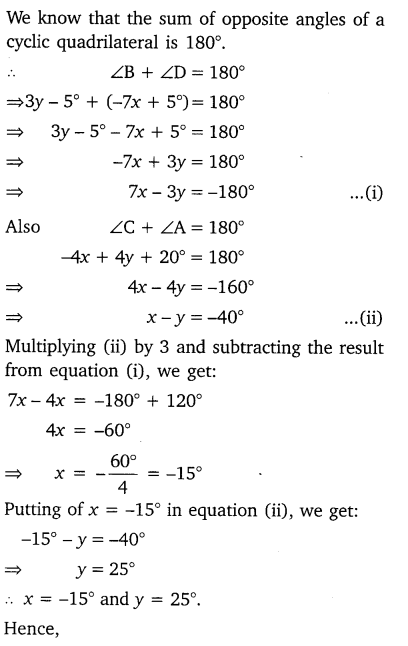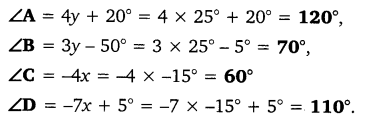We hope the NCERT Solutions for Class 10 Mathematics Chapter 3 Pair of Linear Equations in Two Variables Ex 3.7 help you. If you have any query regarding NCERT Solutions for Class 10 Mathematics Chapter 3 Pair of Linear Equations in Two Variables Ex 3.7, drop a comment below and we will get back to you at the earliest.

## NCERT Solutions for Class 10 Maths Chapter 3 Pair of Linear Equations in Two Variables Ex 3.3

NCERT Solutions for Class 10 Mathematics Chapter 3 Pair of Linear Equations in Two Variables Ex 3.3 are part of NCERT Solutions for Class 10 Maths. Here we have given NCERT Solutions for Class 10 Mathematics Chapter 3 Pair of Linear Equations in Two Variables Ex 3.3. https://mcqquestions.guru/ncert-solutions-for-class-10-maths-chapter-3-ex-3-3/

 Board CBSE Textbook NCERT Class Class 10 Subject Maths Chapter Chapter 3 Chapter Name Pair of Linear Equations in Two Variables Exercise Ex 3.3 Number of Questions Solved 3 Category NCERT Solutions

### NCERT Solutions for Class 10 Maths Chapter 3 Pair of Linear Equations in Two Variables Ex 3.3

Ex 3.3 Class 10 Maths Chapter 3 Question 1.
Solve the following pair of linear equations by the substitution method,
(i) x + y = 14, x – y = 4
(ii) s – t = 3, s/3 + t/2 = 6
(iii) 3x – y = 3, 9x – 3y = 9
(iv) 0.2x + 0.3y = 1.3, 0.4x + 0.5y = 2.3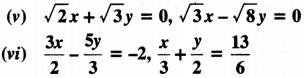Solution:
From equation (i),
x + y – 14 ⇒ y = 14x
Putting the value ofy in equation (ii), we get
x – (14 – x) = 4 ⇒ x – 14 + x = 4 ⇒ 2x = 4 + 14
2x = 18 ⇒ x = 9
Now, puttingx = 9 in equation (i), we have
9 + y = 14 ⇒ y = 14 – 9 ⇒ y = 5
so, x = 9, y = 5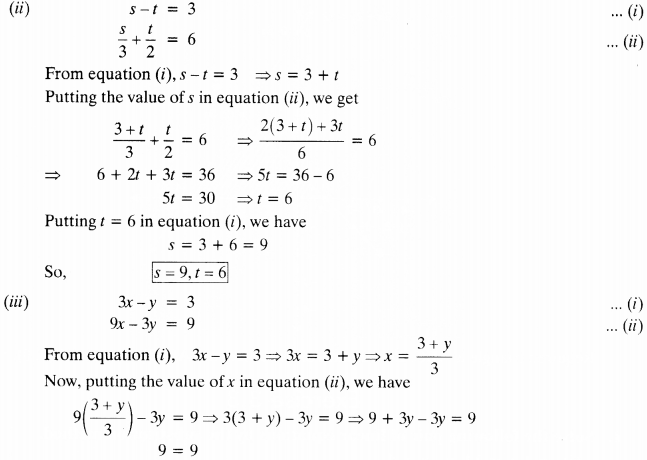∴ y can have infinite real values
∴ x can have infinite real values because x = $$\frac { y+3 }{ 3 }$$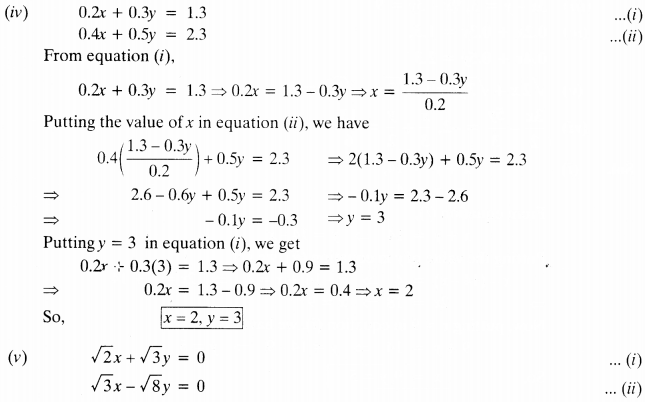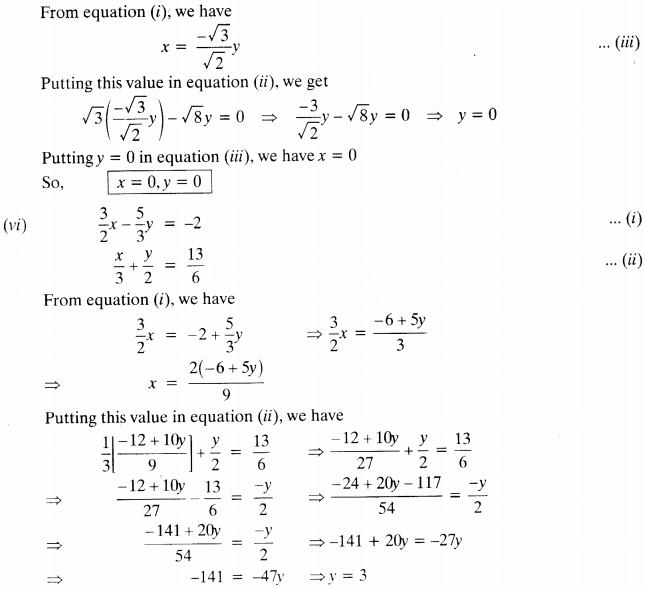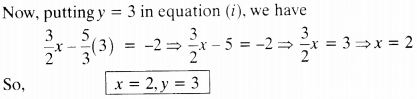Exercise 3.3 Class 10 Maths Question 2.
Solve 2x + 3y = 11 and 2x – 4y = – 24 and hence find the value of ‘m’ for which y = mx + 3.
Solution:
Equations are 2x + 3y = 11
and 2x – 4y = -24
From equation (i)
2x = 11 – 3y
Putting this value in equation (ii), we get
11 – 3y – 4y = -24 ⇒ 11 – 7y = -24 ⇒ – 7y = – 35
y = $$\frac { 35 }{ 7 }$$ ⇒ y = 5
Putting y = 5 in equation (i). we have
2x + 3 x 5 = 11 ⇒ 2x + 15 = 11 ⇒ 2x = 11 – 15 ⇒ 2x = -4 ⇒ x = -2
Now. putting the value of x andy in equation
y = mx + 3 ⇒ 5 = -2m + 3 ⇒ 2 = -2m ⇒ m = -1

3.3 Class 10 Maths Question 3.
Form the pair of linear equations for the following problems and find their solution by substitution method.
(i) The difference between two numbers is 26 and one number is three times the other. Find them.
(ii) The larger of two supplementary angles exceeds the smaller by 18 degrees. Find them.
(iii) The coach of a cricket team buys 7 bats and 6 balls for ₹ 3800. Later, she buys 3 bats and 5 balls for ₹ 1750. Find the cost of each bat and each ball,
(iv) The taxi charges in a city consist of a fixed charge together with the charge for the distance covered. For a distance of 10 km, the charge paid is ₹ 105 and for a journey of 15 km, the charge paid is ₹ 155. What are the fixed charges and the charge per km₹ How much does a person have to pay for travelling a distance of 25 km₹
(v) A fraction becomes 9/2, if 2 is added to both the numerator and the denominator. If, 3 is added to both the numerator and denominator it becomes 5/6, Find the fraction.
(vi) Five years hence, the age of Jacob will be three times that of his son. Five years ago, Jacob’s age was seven times that of his son. What are their present ages?
Solution:
(i) Let 1st number be x and 2nd number be y.
Let x >y
1st condition :
x – y = 26
2nd condition :
x = 3y
Putting x = 3y in equation (i)
3y – y = 26 ⇒ 2y = 26 ⇒ y = 13
From (ii)
x = 3 x 13 = 39
∴ One number is 13 and the other number is 39.

(ii)
Let one angle be x and its supplementary angle = y
Let x > y
1st Condition :
x + y = 180°
2nd Condition :
x – y = 18° ⇒ X = 18° + y
From equation (ii), putting the value ofx in equation (i),
18° + y + y = 180° ⇒ 18° + 2y = 180°
2y = 162° ⇒ y = 81°
From (ii) x = 18° + 81° = 99° ⇒ x = 99°
∴ One angle is 81° and another angle is 99°.

(iii)
Let cost of 1 bat = ₹x and cost of 1 ball = ₹y
1st Condition:
7x + 6y = 3800
2nd Condition:
3x + 5y = 1750
From equation (ii), we get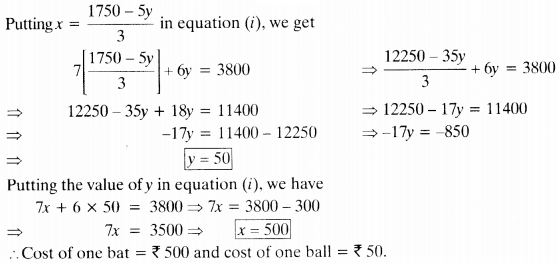putting x = 1750-5y/3 in equation (i), we get
Cost of one bat = ₹ 500 and cost of one ball = ₹ 50.

(iv) Let fixed charges be ₹.v and charge for per km be ₹y.
A.T.Q.
1st Condition :
x + lOy = 105
2nd Condition :
x + 15y = 155
From equation (i), we get
x= 105 – 10y
Putting this value in equation (ii), we have
105 – 10y + 15y = 155 ⇒ 105 + 5y = 155
⇒ 5y = 155 – 105 ⇒ 5y = 50 ⇒ y = 10
Now, puttingy = 10 in equation (i), we have
x + 10(10) = 105 ⇒ x + 100 = 105 ⇒ x = 5
Fixed charges is ₹ 5 and charges per km is ₹ 10.

3rd Condition :
For distance of 25 km
x + 25y = 5 + 25(10) = 5 + 250 = 255
Amount paid for travelling 25 km is ₹ 255.

(v) Let numerator be x and denominator be y.
∴ Fraction is x/y
A.T.Q.
1st condition :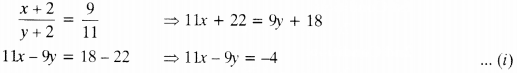2nd condition :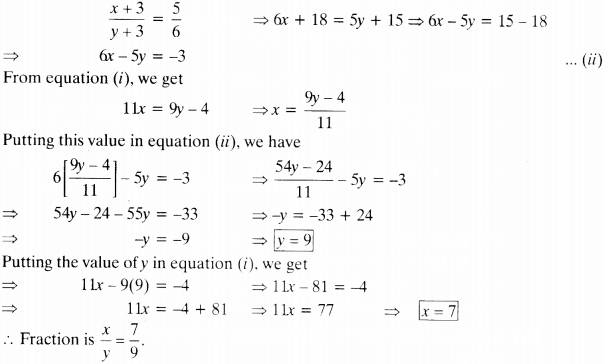(vi) Let present age of Jacob be x years and that of his son bey years.
A.T.Q.
1st Condition :
x + 5 = 3(y + 5) ⇒ x + 5 = 3y + 15 ⇒ x – 3y = 15 – 5 ⇒ x – 3y = 10
2nd Condition:
x – 5 = 7(y – 5) ⇒ x – 5 = 7y – 35 ⇒ x = 7y – 35 + 5
⇒ x = 7y – 30
Putting the value of ‘x’ in equation (i), we get
7y – 30 – 3y = 10
4y – 30 = 10
4y = 40 y = 10 ⇒ y = 10
putting the value of y in equation(ii), we get
x = 7(10) – 30 = 70 – 30 ⇒ x = 40
Hence, the present age of Jacob is 40 years and that of his son is 10 years.

We hope the NCERT Solutions for Class 10 Mathematics Chapter 3 Pair of Linear Equations in Two Variables Ex 3.3 help you. If you have any query regarding NCERT Solutions for Class 10 Mathematics Chapter 3 Pair of Linear Equations in Two Variables Ex 3.3, drop a comment below and we will get back to you at the earliest.

## NCERT Solutions for Class 10 Maths Chapter 1 Real Numbers Ex 1.3

NCERT Solutions for Class 10 Mathematics Chapter 1 Real Numbers Ex 1.3 are part of NCERT Solutions for Class 10 Maths. Here we have given NCERT Solutions for Class 10 Mathematics Chapter 1 Real Numbers Ex 1.3. https://mcqquestions.guru/ncert-solutions-for-class-10-maths-chapter-1-ex-1-3/

 Board CBSE Textbook NCERT Class Class 10 Subject Maths Chapter Chapter 1 Chapter Name Real Numbers Exercise Ex 1.3 Number of Questions Solved 3 Category NCERT Solutions

### NCERT Solutions for Class 10 Maths Chapter 1 Real Numbers Ex 1.3

Exercise 1.3 Class 10 Maths Question 1.
Prove that √5 is irrational.
Solutions:
Let us assume that is rational.
∴ There exists co-prime integers a and b (b ≠ 0) such that
√5 = $$\frac { a }{ b }$$ ⇒ √5b= 0
Squaring on both sides, we get
5b2= a2…… (i)
⇒ 5 divides a2 ⇒ 5 divides a
So, we can write a = 5c for some integer c.
From (i) and (ii)
5b2 = 25c2
⇒ b2 = 5c2
⇒ 5 divides b2
⇒ 5 divides b
∴ 5 is a common factor of a and b.
But this contradicts the fact that a and b are co-primes.
This contradiction has arisen because of our incorrect assumption that √5 is rational.
Hence, √5 is irrational.

1.3 Class 10 Maths Real Numbers Question 2.
Prove that 3 + 2√5 is irrational.
Solutions:
Let us assume that 3 + 2√5 is rational.
∴ There exists co-prime integers a and b(b ≠ 0) such that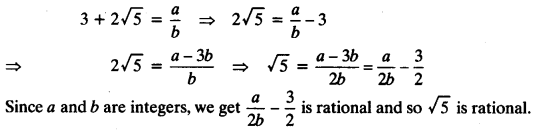But this contradicts the fact that √5 is irrational.
This contradiction has arisen because of our incorrect assumption that 3 + 2√5 is rational. Hence, we conclude that 3 + 2√5 is irrational.

Exercise 1.3 Class 10 Real Numbers Question 3.
Prove that the following are irrationals.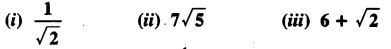Solutions: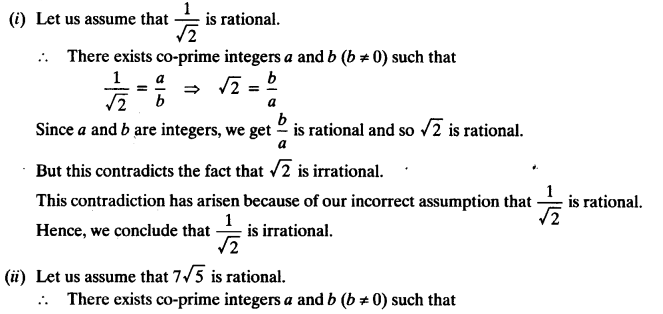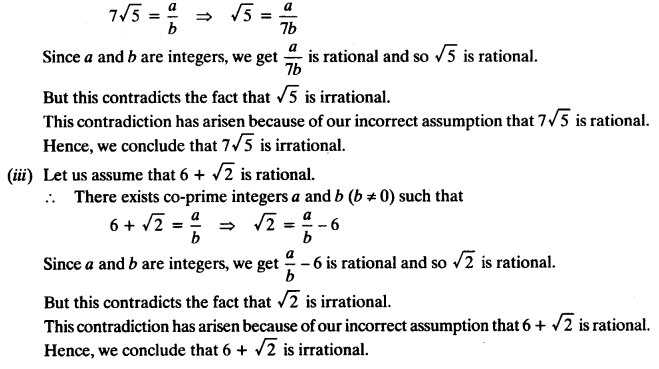We hope the NCERT Solutions for Class 10 Mathematics Chapter 1 Real Numbers Ex 1.3 help you. If you have any query regarding NCERT Solutions for Class 10 Mathematics Chapter 1 Real Numbers Ex 1.3, drop a comment below and we will get back to you at the earliest.